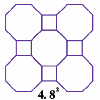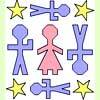#### You may also like### Schlafli Tessellations

are somewhat mundane they do pose a demanding challenge in terms of 'elegant' LOGO procedures. This problem considers the eight semi-regular tessellations which pose a demanding challenge in terms of 'elegant' LOGO procedures.### Napoleon's Theorem

Triangle ABC has equilateral triangles drawn on its edges. Points P, Q and R are the centres of the equilateral triangles. What can you prove about the triangle PQR?### Geomlab

A geometry lab crafted in a functional programming language. Ported to Flash from the original java at web.comlab.ox.ac.uk/geomlab

# Polygon Walk

##### Age 16 to 18 Challenge Level:

My friends Ulaf and Vicky are each given the vector ${\bf i} = (1, 0)$ and another constant vector ${ \bf u}$ and ${\bf v}$ respectively. Starting at the origin, Ulaf and Vicky take a 2-dimensional 'vector walk' where each step is either ${\bf i}$ or one of their constant vectors, either forwards or backwards.

Thus, Ulaf could reach the points $n{\bf i }+m{\bf u}$ and Vicky could reach the points $n{\bf i }+m{\bf v}$ for any whole numbers $n$ and $m$.

After walking around for a while, Ulaf tells me that the points nearest to the origin he could reach formed the vertices of an equilateral triangle and Vicky tells me that the points nearest to the origin that she could reach formed the vertices of a regular hexagon. Using this information, work out ${ \bf u}$ and ${\bf v}$.

Later that day, Wilber went on a vector walk using the vectors ${\bf i} = (1, 0)$ and ${\bf w}$. He tells me that the points nearest to the origin that he could reach formed a regular pentagon. Why must Wilber be mistaken?